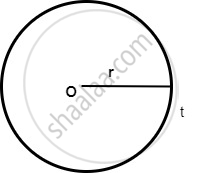SSC (English Medium) Class 9Maharashtra State Board
Share

# Uniform Circular Motion

#### description

• Determining the direction of velocity in uniform circular motion.

#### notes

Uniform Circular Motion:

When an object moves in a circular path with uniform speed, its motion is called uniform circular motion.

In our everyday life, we came across many examples of circular motion for example cars going round the circular track and many more. Also earth and other planets revolve around the sun in a roughly circular orbits

If the speed of motion is constant for a particle moving in a circular motion still the particles accelerates because of constantly changing direction of the velocity.

If an object moves in a circular path with uniform speed, its motion is called uniform circular motion

Here in circular motion, we use angular velocity in place of velocity we used while studying linear motion.

Force which is needed to make body travel in a circular path is called centripetal force.

We know that the circumference of a circle of radius r is given by 2πr. If the body takes t seconds to go once around the circular path of radius r, the velocity v is given by
"v"="2πr"/"t"### Shaalaa.com

Uniform circular motion [00:01:57]
S
series 1
0%

S